Hostname: page-component-cd4964975-96cn4 Total loading time: 0 Render date: 2023-03-30T01:12:47.406Z Has data issue: true Feature Flags: { "useRatesEcommerce": false } hasContentIssue true

# ON IMPROVING ROTH’S THEOREM IN THE PRIMES

Published online by Cambridge University Press:  09 October 2014

## Abstract

Let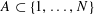$A\subset \{1,\dots ,N\}$ be a set of prime numbers containing no non-trivial arithmetic progressions. Suppose that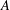$A$ has relative density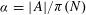${\it\alpha}=|A|/{\it\pi}(N)$, where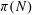${\it\pi}(N)$ denotes the number of primes in the set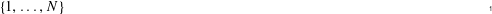$\{1,\dots ,N\}$. By modifying Helfgott and De Roton’s work [Improving Roth’s theorem in the primes. Int. Math. Res. Not. IMRN2011 (4) (2011), 767–783], we improve their bound and show that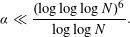$$\begin{eqnarray}{\it\alpha}\ll \frac{(\log \log \log N)^{6}}{\log \log N}.\end{eqnarray}$$

Type
Research Article
Information
Mathematika , January 2015 , pp. 49 - 62

## Access options

Get access to the full version of this content by using one of the access options below. (Log in options will check for institutional or personal access. Content may require purchase if you do not have access.)

## References

Bourgain, J., On triples in arithmetic progression. Geom. Funct. Anal. 9(5) 1999, 968984.CrossRefGoogle Scholar
Bourgain, J., Roth’s theorem on progressions revisited. J. Anal. Math. 104 2008, 155192.CrossRefGoogle Scholar
Erdös, P. and Turán, P., On some sequences of integers. J. Lond. Math. Soc. S1–11(4) 1936, 261264.CrossRefGoogle Scholar
Green, B., Roth’s theorem in the primes. Ann. of Math. (2) 161(3) 2005, 16091636.CrossRefGoogle Scholar
Green, B. and Tao, T., Restriction theory of the Selberg sieve, with applications. J. Théor. Nombres Bordeaux 18(1) 2006, 147182.CrossRefGoogle Scholar
Halberstam, H. and Richert, H.-E., Sieve Methods (London Mathematical Society Monographs 4), Academic Press (London, 1974).Google Scholar
Heath-Brown, D. R., Integer sets containing no arithmetic progressions. J. Lond. Math. Soc. (2) 35(3) 1987, 385394.CrossRefGoogle Scholar
Helfgott, H. A. and de Roton, A., Improving Roth’s theorem in the primes. Int. Math. Res. Not. IMRN 2011(4) 2011, 767783.Google Scholar
Klimov, N. I., Combination of elementary and analytic methods in the theory of numbers. Uspehi Mat. Nauk (N.S.) 13(3(81)) 1958, 145164.Google Scholar
Roth, K. F., On certain sets of integers. J. Lond. Math. Soc. 28 1953, 104109.CrossRefGoogle Scholar
Sanders, T., On Roth’s theorem on progressions. Ann. of Math. (2) 174(1) 2011, 619636.CrossRefGoogle Scholar
Szemerédi, E., Integer sets containing no arithmetic progressions. Acta Math. Hungar. 56(1–2) 1990, 155158.CrossRefGoogle Scholar
van der Corput, J. G., Über Summen von Primzahlen und Primzahlquadraten. Math. Ann. 116(1) 1939, 150.CrossRefGoogle Scholar